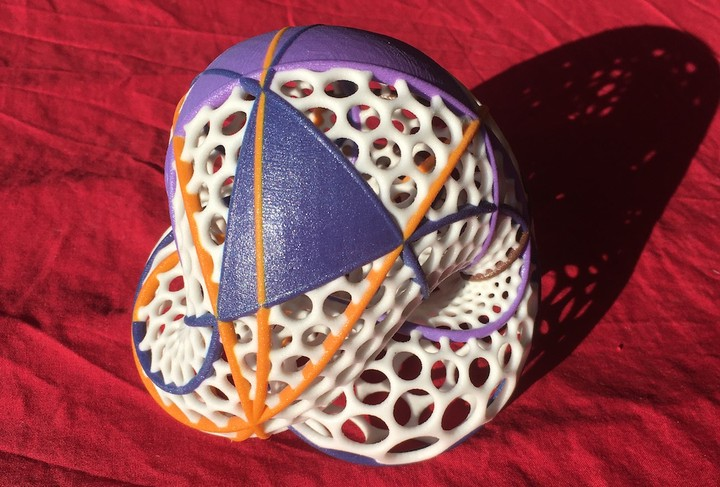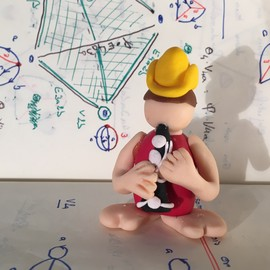# Desargues meets BoyThe Desargues Theorem drawn on a Boy’s surface. Design: R. Coulon

Projective geometry is an important mathematical discovery that goes back to the work of Desargues in the XVIIth century.

## The projective plane

The real projective plane is the space of lines in $\mathbb R^3$ going through the origin. Alternatively it can be obtained for the north hemisphere of the unit sphere in $\mathbb R^3$ by identifying opposite points on the equator. It contains as a subset the usual real plane but cannot be embedded in $\mathbb R^3$.

## The Boy surface

The best we can hope for is an immersion of the real projective plane in $\mathbb R^3$. This is precisely what the Boy surface is. Invented by Werner Boy there are several parametrization of this surface. The one pictured below is attributed to F. Apery.Apery’s parametrization of the Boy surface. 3D printed model. Design: R. Coulon

Another parametrization, due to Bryant and Kusner has the property to be the “least bend” immersion of the Boy surface. Below a bronze model built from this parametrization.Bryant-Kusner’s parametrization of the Boy surface. Bronze cast. Design: R. Coulon

This parametrization is famous among mathematicians, since it is the one welcoming the visitors at the Mathematisches Forschungsinstitut Oberwolfach

## The Desargues Theorem

Start with two triangles in the real projective plane, with vertices say $A_1$, $A_2$, $A_3$ and $B_1, B_2, B_3$ in the projective plane. Consider now the following two questions:

• Question 1. Do the lines $(A_1B_1)$, $(A_2B_2)$ and $(A_3B_3)$ have a common intersection point?
• Question 2. Denote by $C_1$ – respectively $C_2$, $C_3$ — the intersection point of $(A_2A_3)$ and $(B_2B_3)$ — respectively $(A_3A_1)$ and $(B_3B_1)$, $(A_1A_2)$ and $(B_1B_2)$. Do the points $C_1$, $C_2$ and $C_3$ lie on the same line?

Desargues’ Theorem states that the answer to Question 1 is “Yes” if and only if the answer to Question 2 is “Yes” as well. The figure below represents a configuration of the Desargues theorem in the affine plane (i.e. when the two questions have a positive answer).

The triangles are represented in blue and purple. The lines $(A_1B_1)$, $(A_2B_2)$ and $(A_3B_3)$ are yellow. The points $C_1$, $C_2$ and $C_3$ lies on the brown line.